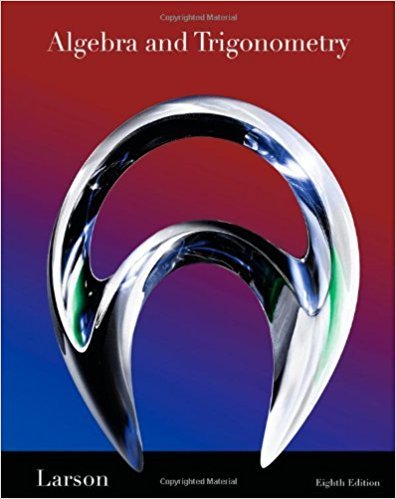×
×

# Solutions for Chapter 11.7: Probability## Full solutions for Algebra and Trigonometry | 8th Edition

ISBN: 9781439048474Solutions for Chapter 11.7: Probability

Solutions for Chapter 11.7
4 5 0 402 Reviews
28
0
##### ISBN: 9781439048474

This textbook survival guide was created for the textbook: Algebra and Trigonometry, edition: 8. Since 76 problems in chapter 11.7: Probability have been answered, more than 51787 students have viewed full step-by-step solutions from this chapter. This expansive textbook survival guide covers the following chapters and their solutions. Chapter 11.7: Probability includes 76 full step-by-step solutions. Algebra and Trigonometry was written by and is associated to the ISBN: 9781439048474.

Key Math Terms and definitions covered in this textbook
• Characteristic equation det(A - AI) = O.

The n roots are the eigenvalues of A.

• Column picture of Ax = b.

The vector b becomes a combination of the columns of A. The system is solvable only when b is in the column space C (A).

• Cyclic shift

S. Permutation with S21 = 1, S32 = 1, ... , finally SIn = 1. Its eigenvalues are the nth roots e2lrik/n of 1; eigenvectors are columns of the Fourier matrix F.

• Diagonalizable matrix A.

Must have n independent eigenvectors (in the columns of S; automatic with n different eigenvalues). Then S-I AS = A = eigenvalue matrix.

• Diagonalization

A = S-1 AS. A = eigenvalue matrix and S = eigenvector matrix of A. A must have n independent eigenvectors to make S invertible. All Ak = SA k S-I.

• Ellipse (or ellipsoid) x T Ax = 1.

A must be positive definite; the axes of the ellipse are eigenvectors of A, with lengths 1/.JI. (For IIx II = 1 the vectors y = Ax lie on the ellipse IIA-1 yll2 = Y T(AAT)-1 Y = 1 displayed by eigshow; axis lengths ad

• Fibonacci numbers

0,1,1,2,3,5, ... satisfy Fn = Fn-l + Fn- 2 = (A7 -A~)I()q -A2). Growth rate Al = (1 + .J5) 12 is the largest eigenvalue of the Fibonacci matrix [ } A].

• Fourier matrix F.

Entries Fjk = e21Cijk/n give orthogonal columns FT F = nI. Then y = Fe is the (inverse) Discrete Fourier Transform Y j = L cke21Cijk/n.

• Hessenberg matrix H.

Triangular matrix with one extra nonzero adjacent diagonal.

• Markov matrix M.

All mij > 0 and each column sum is 1. Largest eigenvalue A = 1. If mij > 0, the columns of Mk approach the steady state eigenvector M s = s > O.

• Multiplier eij.

The pivot row j is multiplied by eij and subtracted from row i to eliminate the i, j entry: eij = (entry to eliminate) / (jth pivot).

• Partial pivoting.

In each column, choose the largest available pivot to control roundoff; all multipliers have leij I < 1. See condition number.

• Projection p = a(aTblaTa) onto the line through a.

P = aaT laTa has rank l.

• Pseudoinverse A+ (Moore-Penrose inverse).

The n by m matrix that "inverts" A from column space back to row space, with N(A+) = N(AT). A+ A and AA+ are the projection matrices onto the row space and column space. Rank(A +) = rank(A).

• Rank one matrix A = uvT f=. O.

Column and row spaces = lines cu and cv.

• Saddle point of I(x}, ... ,xn ).

A point where the first derivatives of I are zero and the second derivative matrix (a2 II aXi ax j = Hessian matrix) is indefinite.

• Spectral Theorem A = QAQT.

Real symmetric A has real A'S and orthonormal q's.

• Toeplitz matrix.

Constant down each diagonal = time-invariant (shift-invariant) filter.

v + w = (VI + WI, ... , Vn + Wn ) = diagonal of parallelogram.

• Vector space V.

Set of vectors such that all combinations cv + d w remain within V. Eight required rules are given in Section 3.1 for scalars c, d and vectors v, w.

×